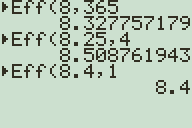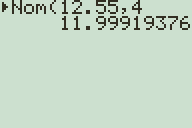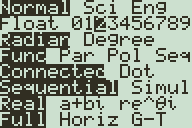# Business and Finance Math #5: Calculating the Effective Annual Rate (EAR) on the TI-83 & TI-84 Plus

Filed under Difficulty: Easy, Finance, TI-83 Plus, TI-84 Plus.

While they are intended primarily for math and statistics work, the TI 83 Plus and TI 84 Plus also have a slew of built in financial functions similar to those available on advanced financial calculators. This tutorial describes how to use the EAR (effective annual rate) and NOM (nominal rate) functions of your calculator to solve the problems in Financial Math Tutorials 2 and 3, which covered conversion between effective annual rates and stated interest rates.

In Business and Finance Math #2: Calculating the Effective Annual Rate (EAR) on Your TI BA II Plus or HP 12c, we covered the basic concept and method for calculating EAR mathematically and used it to solve the problem of which of the following interest rates was better:

• 8% compounded daily
• 8.25% compounded quarterly
• 8.4% compounded annually

To reach the Finance menu on your TI-83 Plus or TI-84 Plus, press to select the applications menu. “Finance…” should be the top option, so press to select.Use the button to select ▶Eff( from the finance menu, and press to paste it to the home screen.Enter the interest rate in percent, press and enter the number of compoundings per year, and press to calculate the effective annual rate for the interest rate you entered.The calculator tells us that the EAR of 8.25% compounded quarterly is about 8.51%, the highest of the 3 possible options.

In Business and Finance Math #3: Converting Effective Interest Rates (EAR) to Stated Interest Rates, we discussed the inverse problem, how to convert an EAR to an interest rate with a certain number of compoundings per year. The given problem was to convert an EAR of 12.55% to a stated interest rate compounded quarterly.

To perform this operation on the TI-83 Plus or TI-84 Plus, select ▶Nom( from the finance menu and press to paste it to the home screen. (The nominal rate function is right about the effective rate function on the menu.) Then enter 12.55 for the effective rate, to separate arguments, and 4 for the number of compoundings annually. Press to compute.You may notice that your calculator displays many unnecessary decimals. To fix this, press to enter the Calculator Mode menu, and select the number of decimal places you want to have, or FLOAT if you want as many decimal places as the calculator can handle.Now performing the same calculation displays a rate of 12% exactly because it rounds every answer to two decimal places. If you work with money and percentages, then you may not need more than two or three decimal places, but remember that this will affect all other calculations you make.We also have an EAR program that doesn’t need your calculator’s FINANCE apps installed here. Be sure to read the previous Business and Finance Math articles to better understand the mathematics behind effective and stated interest rates. If you have any further questions or other topics you would like to see articles on, be sure to leave a comment or drop us an email through our contact form.